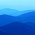## Friday, 28 June 2013

### Video Solutions for GATE 1990 ECE : One Mark Questions ( Electron Devices )

1.       Under high electric fields, in a semiconductor with increasing
electric field,

a. the mobility of charge carriers decreases

b. the mobility of charge carriers increases

c. the velocity of charge carriers saturates

d. the velocity of charge carriers increases

2.       In a uniformly doped abrupt PN junction, the doping level of
the N-side is four times the doping level of the P-side.
The ratio of the depletion layer widths is

a.       0.25

b.       0.50

c.        1.0

d.       2.0

3.       In a forward biased photo diode, with increase in incident
light intensity, the diode current

a.       Increases

b.      Remains constant

c.       Decreases

d.      Remaining constant, but the voltage drop across the diode increases.

4.       In a junction diode,

a.       The depletion capacitance increases with increase in the reverse bias

b.      The depletion capacitance increases with decrease  in the reverse bias

c.       The diffusion capacitance increases with increase in the forward bias

d.      The diffusion capacitance is much higher than the depletion capacitance, when its is forward biased

5.       SCR can be turned on by

a.       Applying anode voltage at a sufficiently fast rate

b.      Applying sufficiently large anode voltage

c.       Increasing the temperature of SCR to a sufficiently large value

d.      Applying sufficiently large gate current6.       The pinch off voltage of a JFET is 5.0 volts. Its cutoff voltage
is

a.       (5.0)1/2 volts

b.      2.5 volts

c.       5.0 volts

d.      (5.0)3/2 volts

7.       Which of the following effects can be caused by a rise in the
temperature ?

a.       Increase in MOSFET current (IDS)

b.      Increase in BJT current (IC)

c.       Decrease in MOSFET current (IDS)

d.      Decrease in BJT current (IC)

1.2.3.